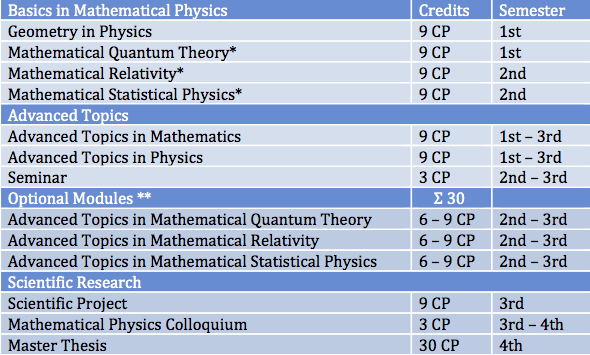Department of Mathematics

# Program structure

Details on the study program are described in the Module Handbook. Here we give only a short summary of the overall structure.

The master's program consists of the following components:* Two out of the three basic modules Mathematical Quantum Theory, Mathematical Relativity, and Mathematical Statistical Physics are mandatory. The third one is optional.

All other courses can be chosen according to personal preferences with one limitation: every student has to pick at least one course from Mathematics (i.e. the module Advanced Topics in Mathematics) and one course from Physics (i.e. the module Advanced Topics in Physics).

** Within the area "Optional Modules", the three listed modules from the Mathematical Physics program can be chosen as well as a large number of advanced modules of the master degree programs Mathematics, Physics, or Astro and Particle Physics. Typical choices from Mathematics would be partial differential equations, numerical analysis of differential equations, harmonic analysis, Lie groups, nonlinear functional analysis, operator theory, stochastic processes, calculus of variations, symplectic geometry, algebraic topology, and algebraic geometry. Typical choices from Physics would include quantum field theory and particle physics, theoretical astrophysics, relativistic astrophysics, advanced statistical physics, astronomy & astrophysics, theoretical condensed matter physics, theoretical quantum optics, quantum information theory, cosmology, computational methods in physics/astrophysics.

Note that some modules in the master degree programs Mathematics and Physics may sometimes be offered in German only and thus choices could be slightly restricted for students not speaking German.

The Mathematical Physics Colloquium is a weekly colloquium where specialists lecture about recent developments in Mathematical Physics. This provides students with the opportunity to meet and discuss with international guest scientists and local professors about current research and thereby to practice scientific discourse. Moreover, every student will present the results of his or her own master thesis research within the Mathematical Physics Colloquium.

The master thesis is supervised by two professors, ideally one from Mathematics and one from Physics.

## Mentoring

Every student will be assigned a mentor from the group of professors involved in the master's program. In particular, the choice of modules for each semester must be discussed with and approved by the mentor. This ensures that students choose matching modules in line with their desired specialization.